# The Formula Column

Missing the Excel formula and mathematic capabilities? Not to worry! We came up with the formula column just for this. This column allows you to make calculations with other columns. It goes from simple mathematical equation to more complex functions and formulas.Note:  The formula column is only available for the Pro and Enterprise plan. If you were on a Pro plan and downgraded your account, the formula columns you have created will remain intact, but you will not be able to add more formula columns.

To add it, click on the + icon located on the top right of your board and select "column center". Once in the center, select the option "Formula" as in the screenshot below: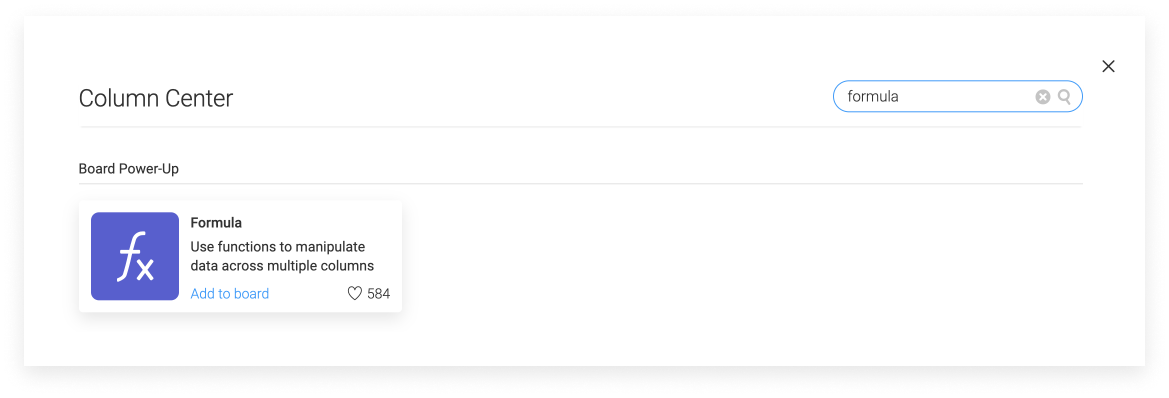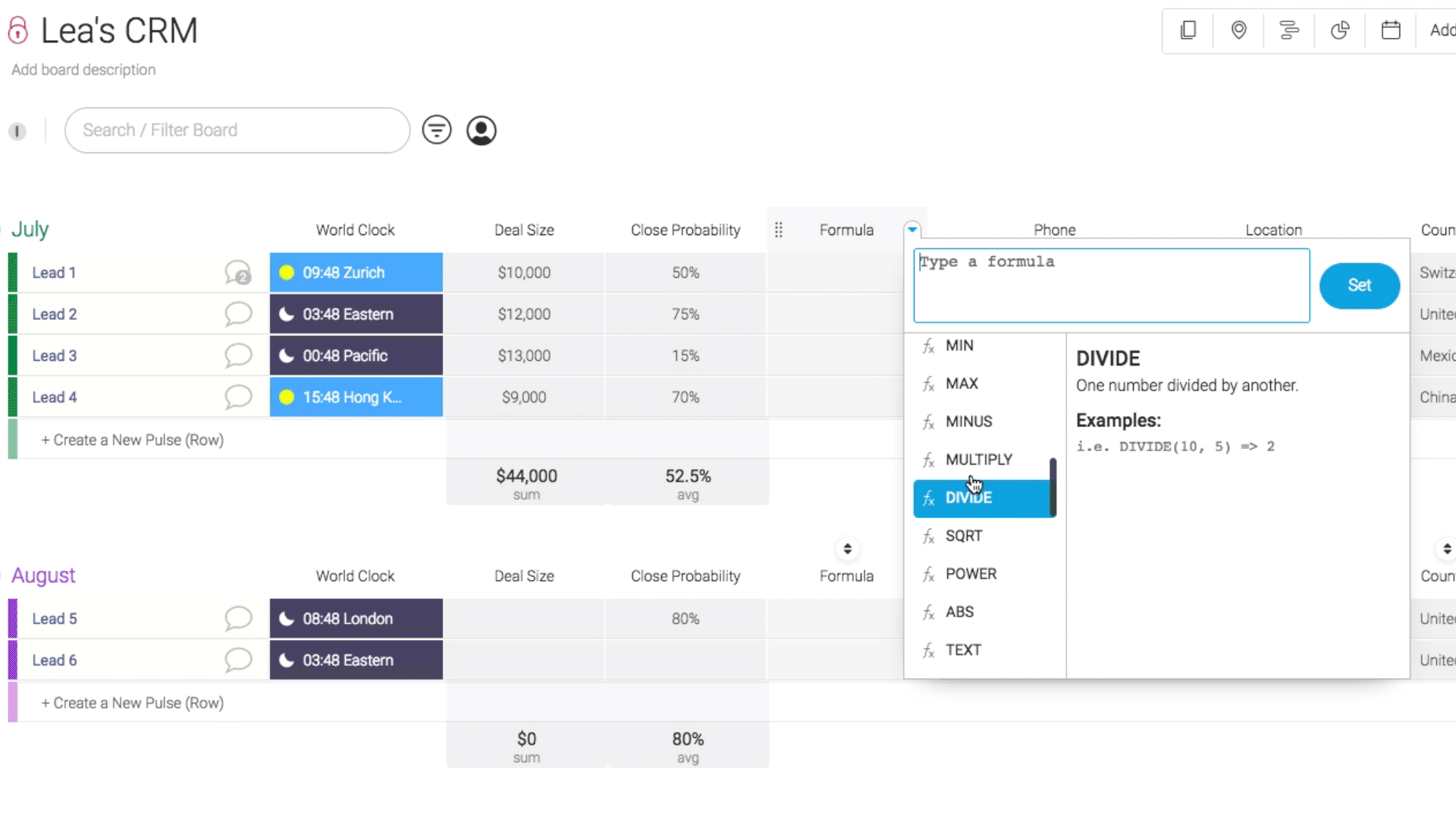# Can I format my formula?

You can format your result exactly the way you want it to look like by using the formula TEXT. Let's take an easy example: I have want to multiply 10 by 1250 and I want my number to show the following way: \$12,500.00.Formula:  TEXT(MULTIPLY({Deals},{Amount}),"\$#,##.00")Each # represents a number. I have added .00 by the end because I want my formula to end by .00 but you can replace this by .## if you want your number to end with proper numbers.

# Add a total at the bottom of the formula

Exactly like for the numbers column, you can see the overall calculation for each group.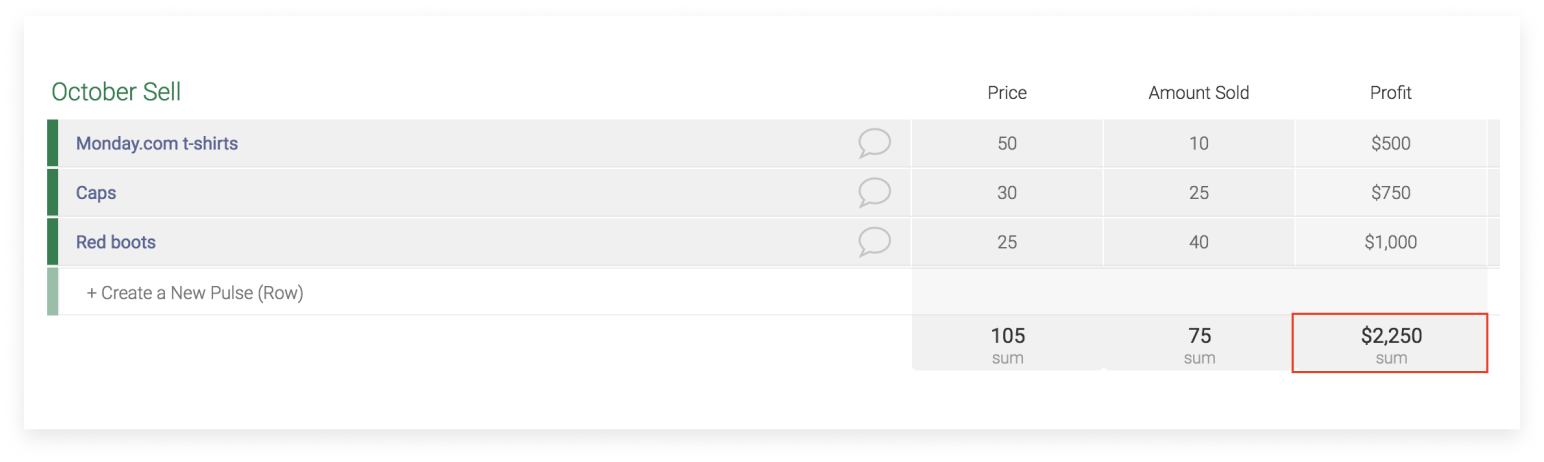By clicking on this box, you will also be able to change the unit and the function as below: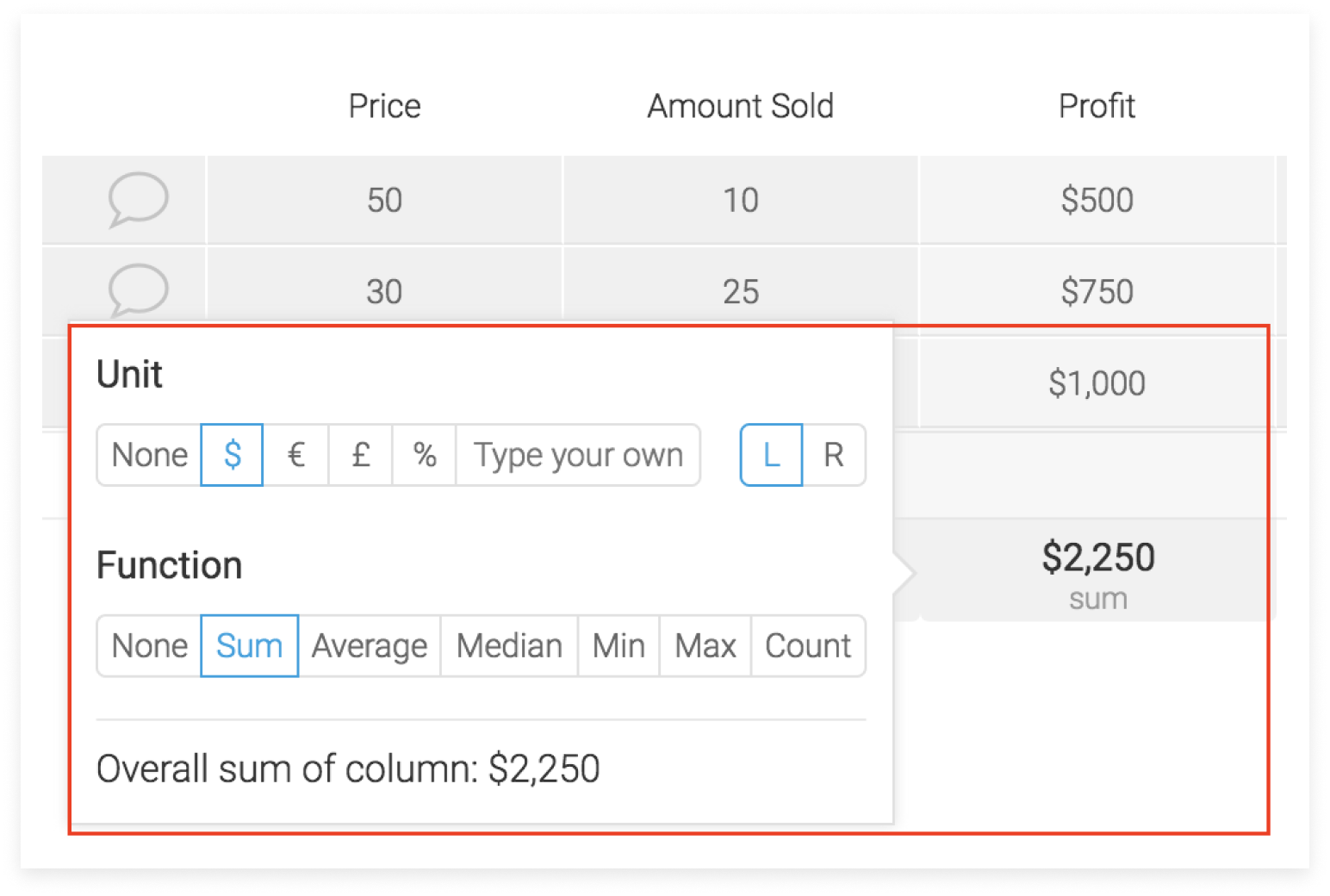Note: The total only takes into account numeric values. If your column has both numeric and text values, we will only count the numeric values. Mixed values like Lea123 will not be taken into account in the total. If all values are non-numeric, you will get N/A at the bottom as in the screenshot below.

# Use CasesImportant Tip: To make it easier for you, we have built a monday board with all our use cases. Click here to check it out.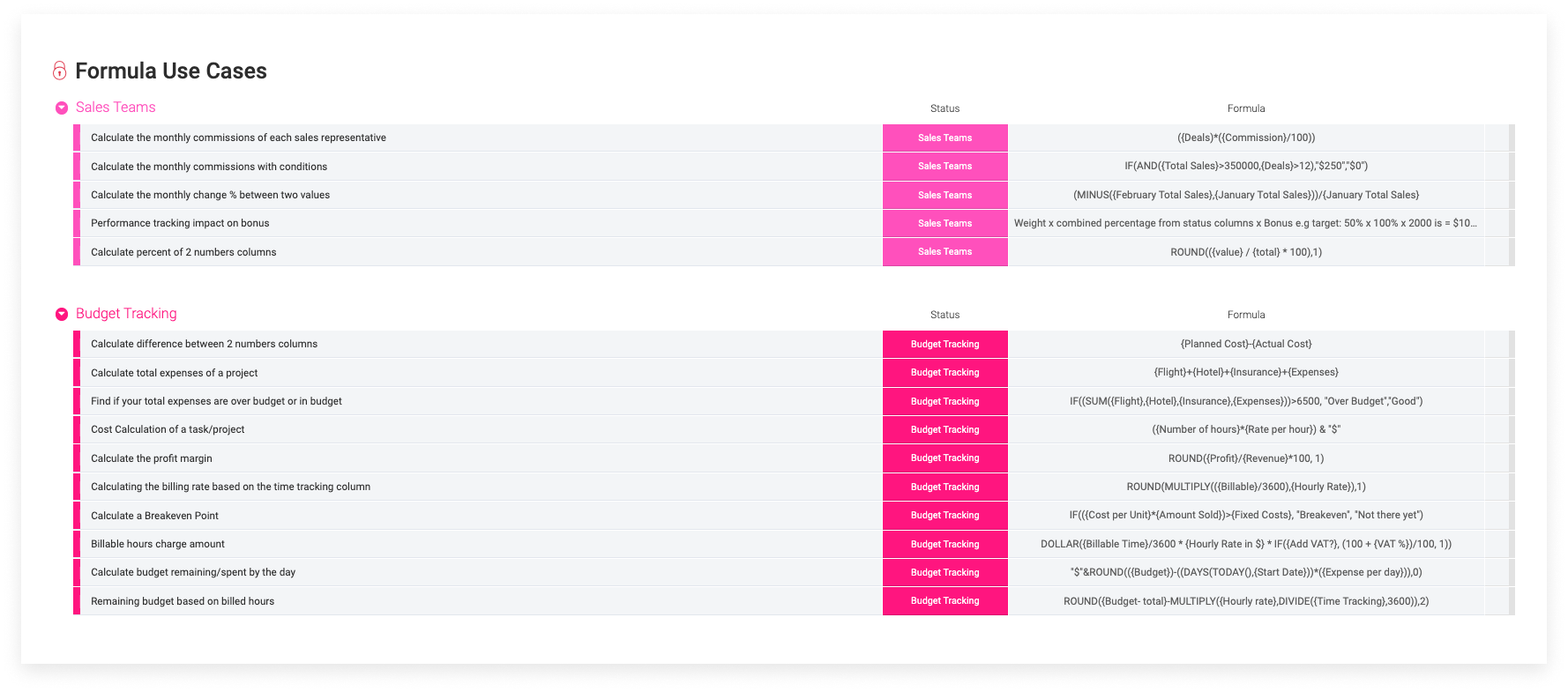Below are some of the popular use cases:

• Calculate the monthly commissions with conditions

Calculate if your employees are entitled to get a bonus. An employee can get a bonus only IF the "total sales" is higher than \$350,000 AND IF the amount of deals is higher than 12.Formula: IF(AND({Total Sales}>350000,{Deals}>12),"\$250","\$0")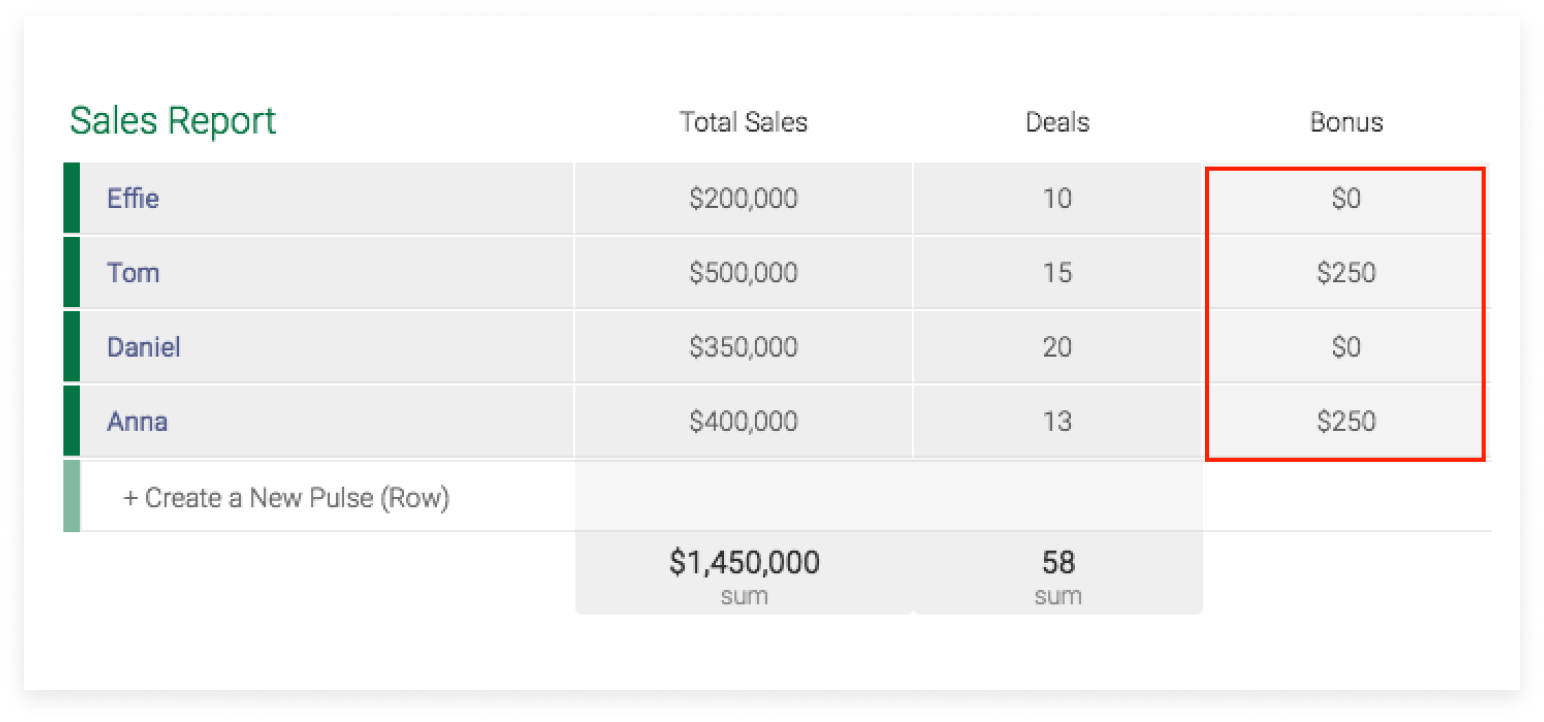• Calculate the monthly change % between two values

I want to calculate the monthly change between the results of January and February.Formula: (MINUS({February Total Sales},{January Total Sales}))/{January Total Sales}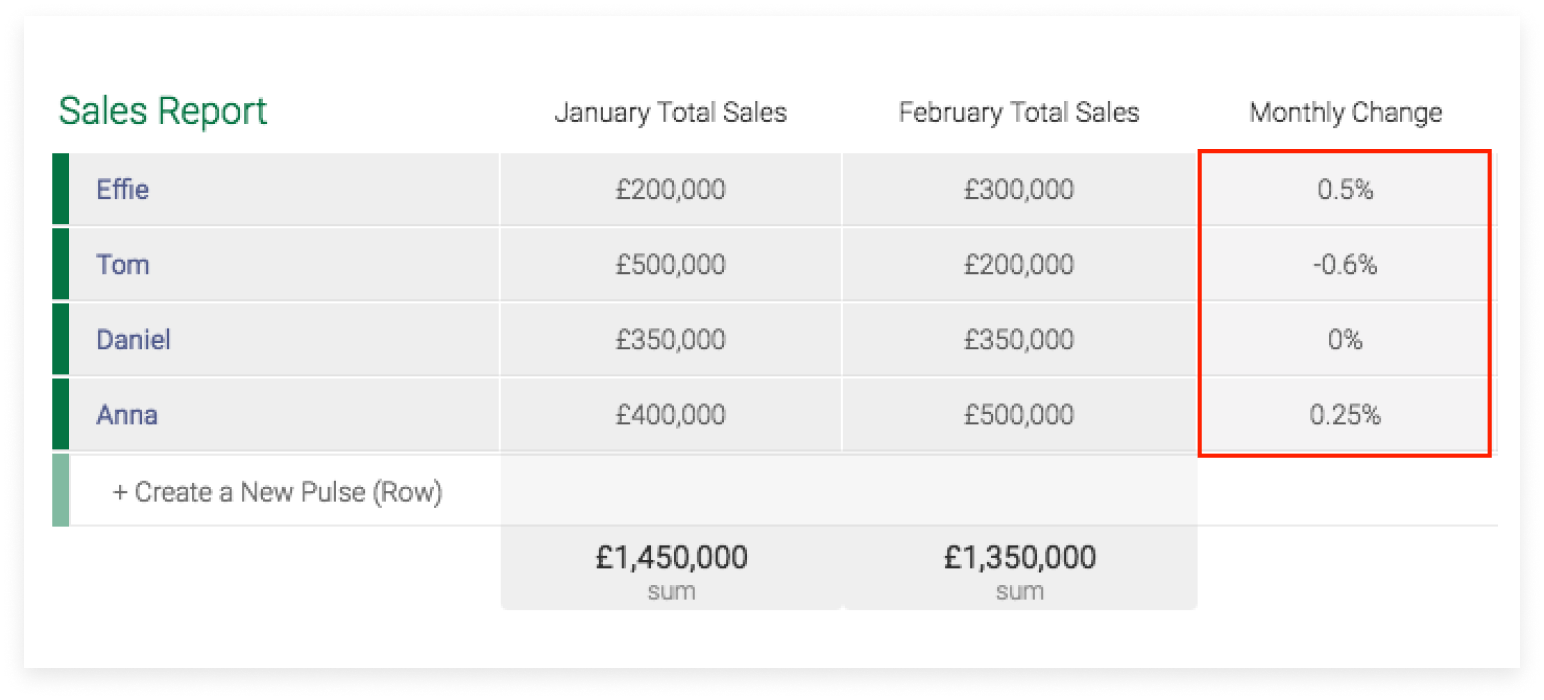• Find if your total expenses are over budget or in budget

My budget is \$6500 per employee. I want to find out if the total amount I spent for each employee is in the budget or over it.Formula: IF((SUM({Flight},{Hotel},{Insurance},{Expenses}))>6500, "Over Budget","Good")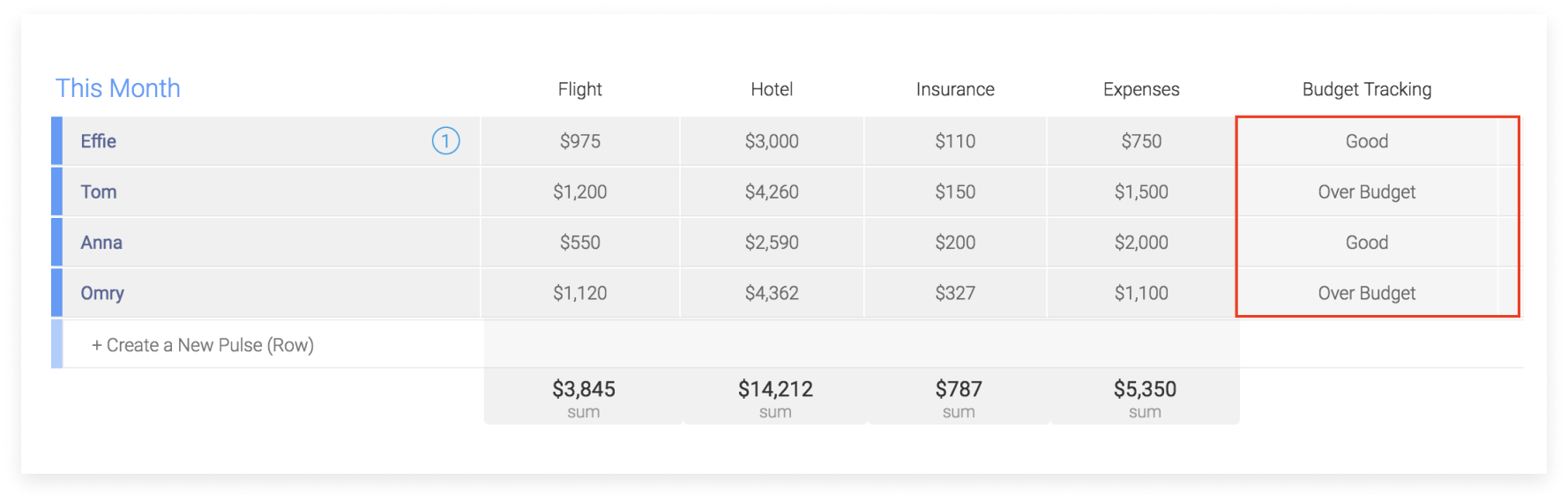• Calculating the billing rate based on the time tracking columnFormula: ROUND(MULTIPLY(({Billable}/3600),{Hourly Rate}),1)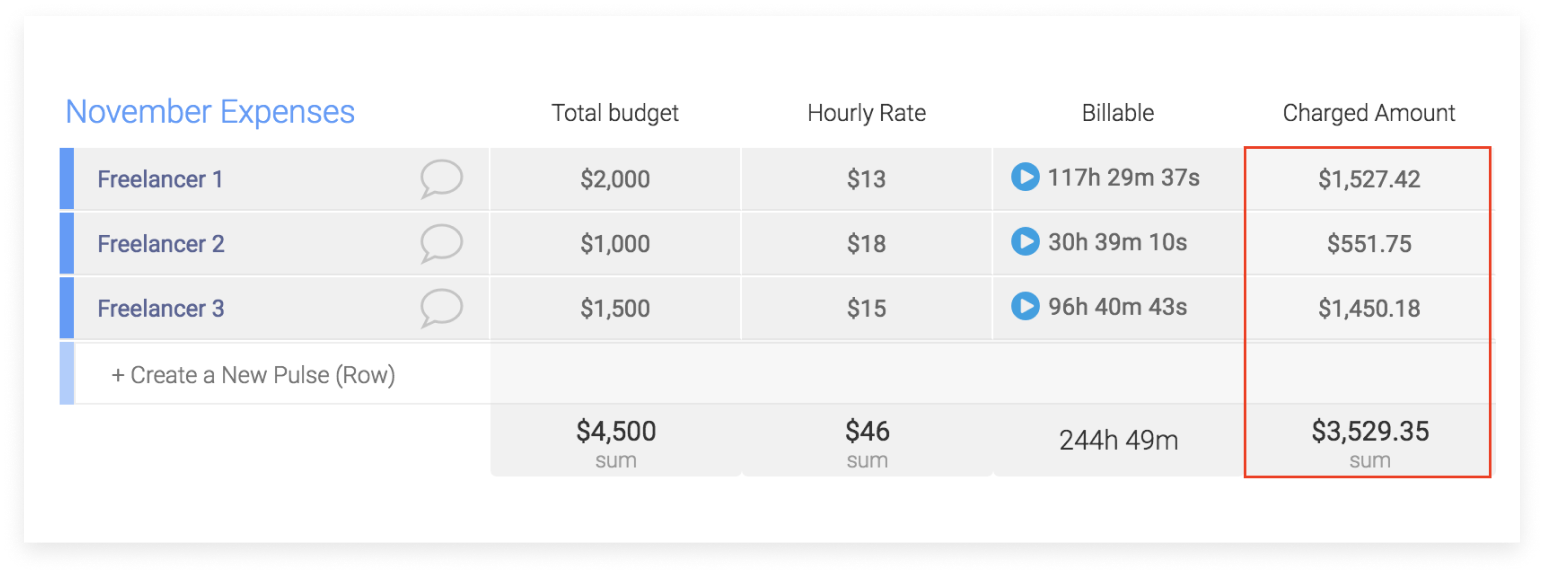• Keep Track of your inventory

You can easily manage your inventory with monday.com. This example explains you how to calculate your current available stock and how much were sold in percentage.Formula: MINUS({Purchased},{Reserved},{Sold})Formula: ROUND({Sold}/{Purchased}*100,2)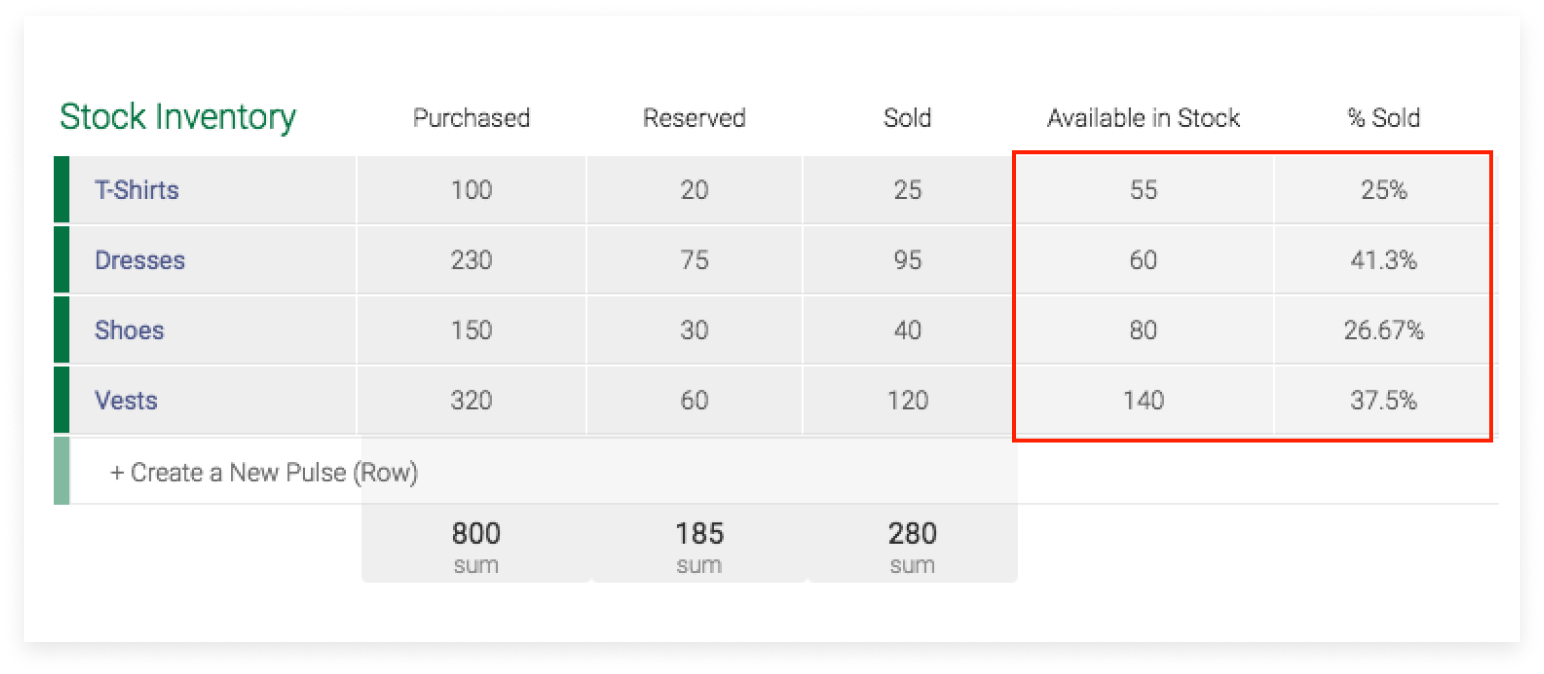• Get numbers of days between two dates excluding the weekends

At monday.com, we are using a vacation board to keep track of everyone's holidays. We use the formula column to see how many working days each team member will be missing.Formula: DAYS({To},{From})-(2*(WEEKNUM({To})-WEEKNUM({From})))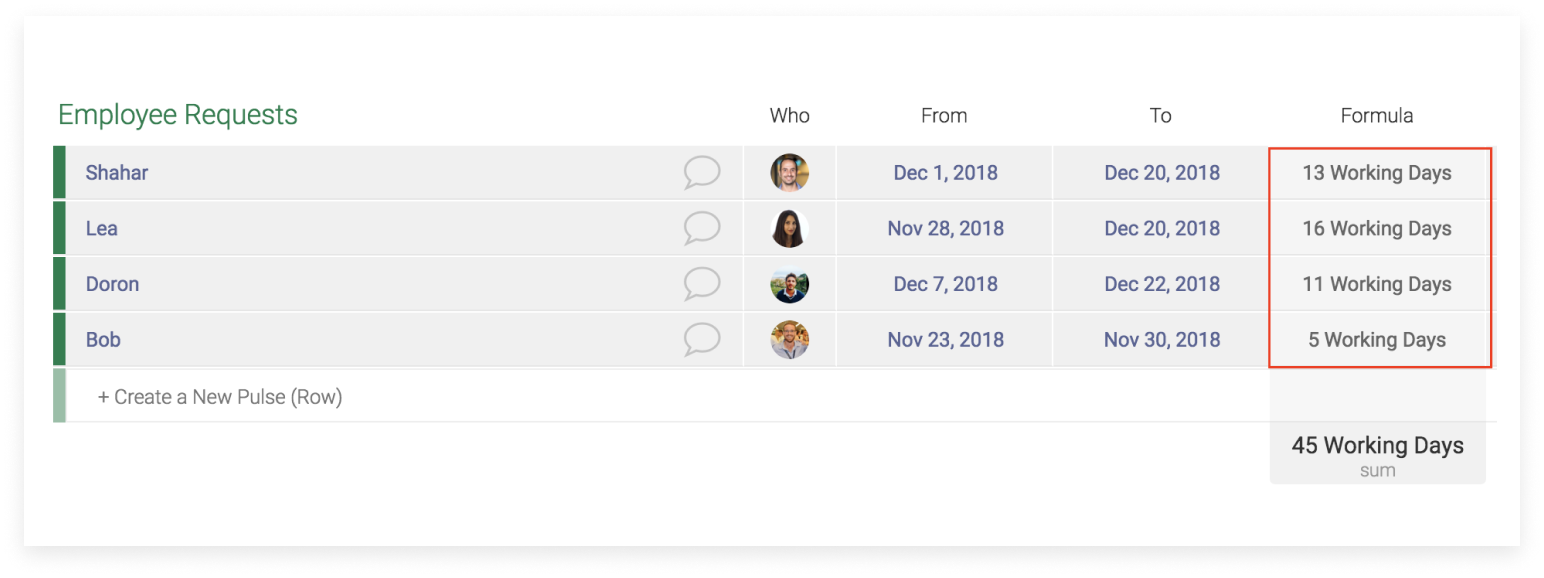• Get the countdown between today and the due date of your project

I want to know how many days remain until the due date of my task.Formula: ROUND(DAYS({Due Date},TODAY()),0)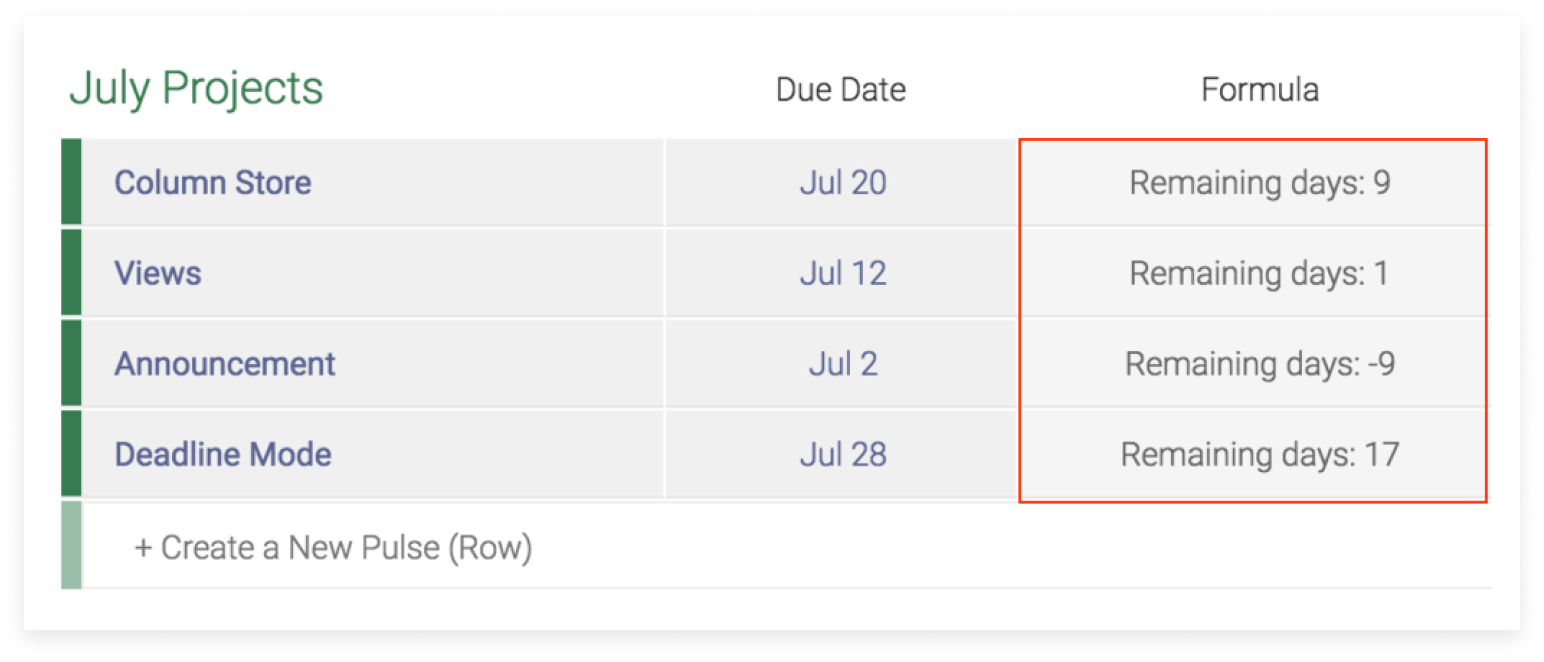• Adding/Subtracting days to a date

In this example, we want to add 15 days to each date from the column "start date". This first formula will show you a result unformatted which might look a little clunky.Formula: (DATE(YEAR({Start Date}),MONTH({Start Date}),DAY({Start Date})+15))

This is why we recommend to use the below formula. The result is formatted and will look cleaner in your board.Formula: RIGHT(LEFT(CONCATENATE(DATE(YEAR({Start Date}),MONTH({Start Date}),DAY({Start Date})+15)),10),6)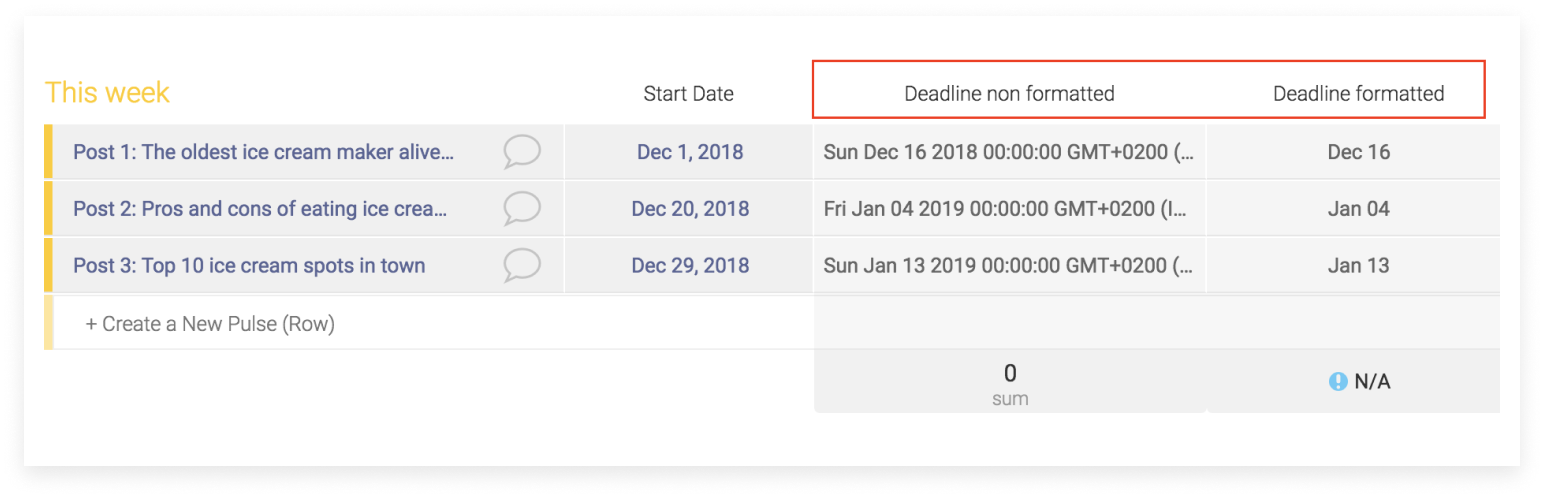• Use Status labels to assign commission rates

In this example, let's say I manage a sales team with varying commission rates per project or salesperson.  You can use the labels from the status column to manipulate the formula to calculate different rates based on which label is selected:Formula: IF({Agent}="Agent 1",15,IF({Agent}="Agent 2",20,IF({Agent}="Agent 3",12)))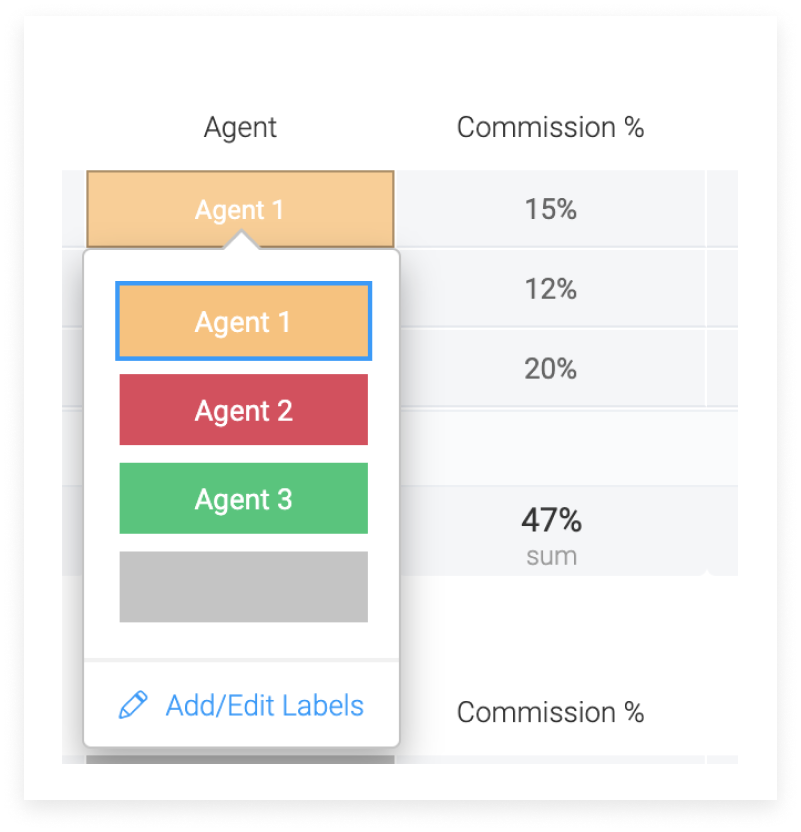• Calculating how long somebody worked, subtracting their break

In this example, I'd like to calculate how long my employees have worked, minus their unpaid break time.  Using four "Hour" columns, I can create a formula to calculate just this:Formula: IF((HOURS_DIFF({Break End}, {Break Start})) > "0", (HOURS_DIFF((HOURS_DIFF({End}, {Start})), (HOURS_DIFF({Break End}, {Break Start})))), (HOURS_DIFF({End}, {Start})))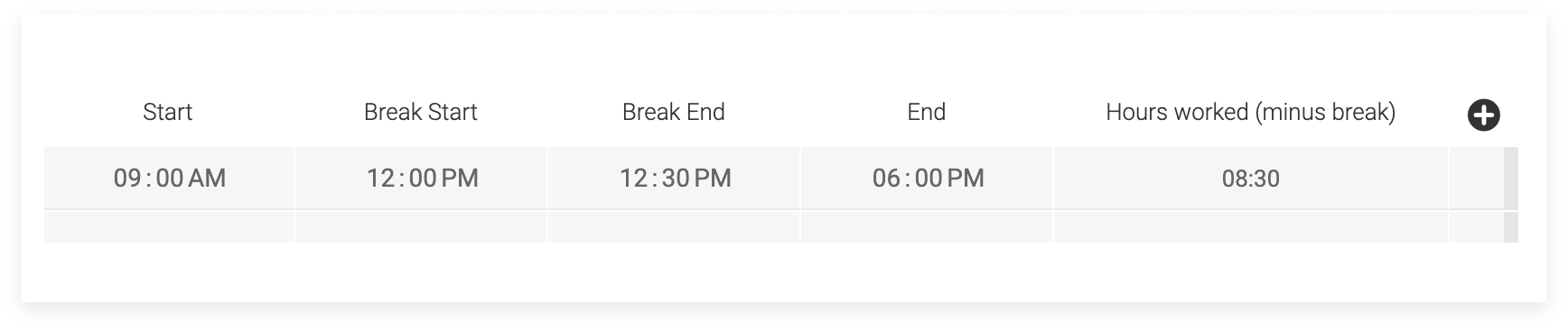If you have any further questions about the formula column please don't hesitate to reach out to our customer success team at [email protected]